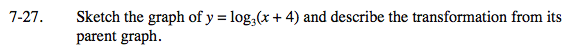Home > INT3 > Chapter 7 > Lesson 7.1.2 > Problem7-27

7-27.

Sketch the graph of y = log3(x + 4) and describe the transformation from its parent graph. Homework Help ✎Use the log function for the parent graph.

Make a sketch of the graph y = log3(x). This graph is shifted four units to the left. Move each point on the graph of y = log3(x) four units to the left. Since the vertical asymptote of the parent graph was x = 0, for this graph it should be x = −4.